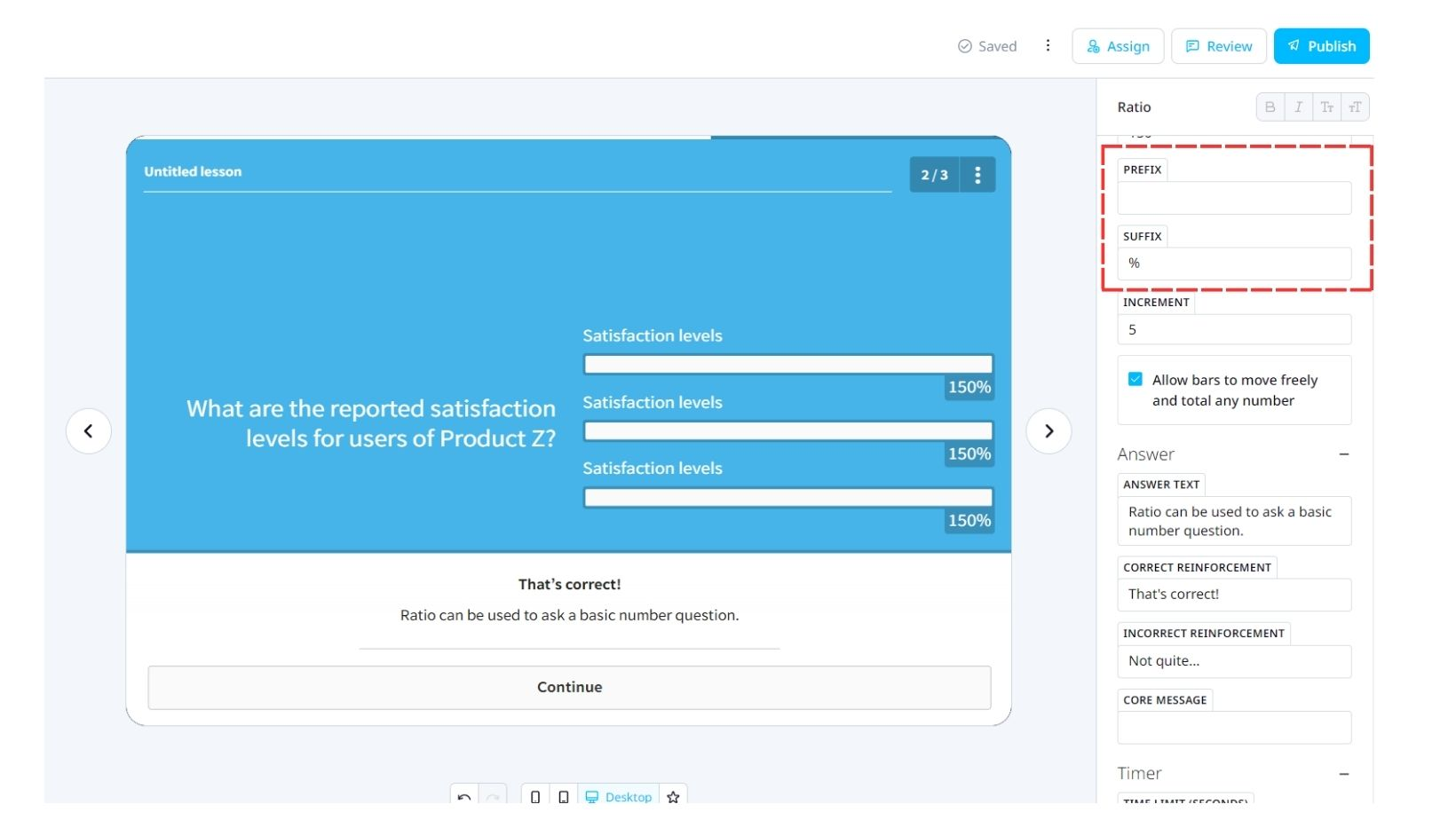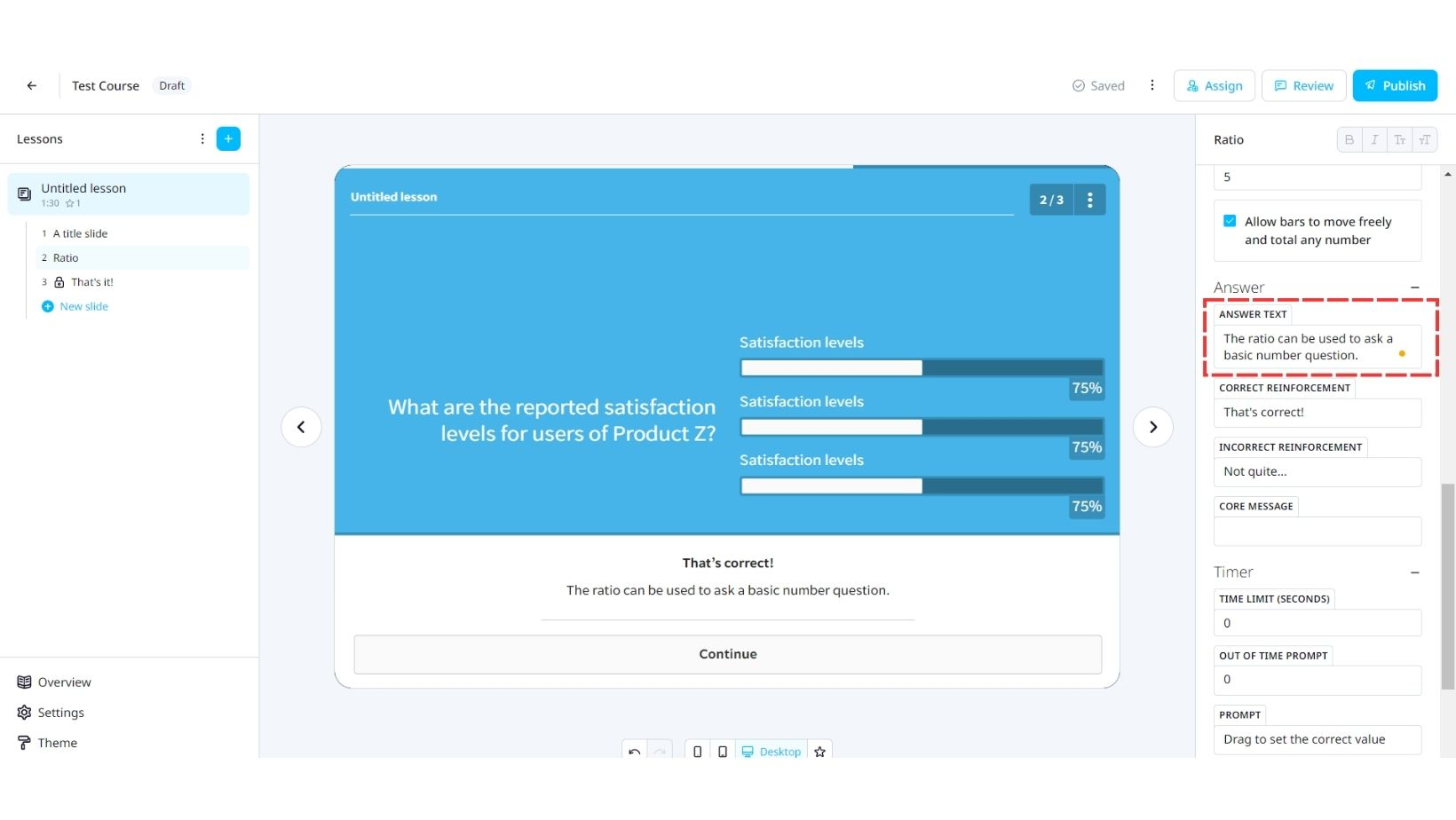# Ratio

## EdApp's Ratio template is the perfect way to create comparisons in learning.

Key Takeaway: Not just used for percentages, Ratio can be used to create comparisons between the weight and price of products. A step-by-step guide to customizing the Ratio template.

The EdApp template Ratio has a variety of applications. The great thing about EdApp is that each of our templates can be customized for a variety of functions.

Ratio, not only asks your users to identify ratios and percentages but can also be utilized in your mobile learning to create monetary, volume, or weight comparisons for your users.

In this article, we will go through applying a Ratio template to a lesson step-by-step from within the creator tool.

### 1. Title

Set the title or question you would like answered in the Title field.

### 2. Bars

Under the Bars tab, you can set the item names for each of your bars and their correct value.

The Total Value of all Bars is the total value you would like the amount to be set at or alternatively you can set the length of each bar. Users indicate their answer by sliding the bar to a ratio of this value, therefore the Total Value of All Bars must be equal to or greater than that of the highest value.

In this example, we have set the Total Value of all Bars to 150. That way, users can see satisfaction levels in a comparative view and visually compare and relate each Satisfaction Level to answer the question.

### 3. Prefix/Suffix

Typically, the value identifier for each bar is a percentage. However, as you can see here, you can customize the template to your individual need. Simply add a prefix (such as currency) or a suffix (for weight, length, etc.)Additional features are available on the Ratio enabling you to further customize this template if required.

Allow bars to move freely
– By default, the bars move in relation to each other. For example, if one bar is set to 20% then the other two will accommodate to equal a sum of 80%. You can Allow bars to move freely if you wish users to answer each independently as seen in this example.

Increment – Here you can set the increment value you wish numbers to increase/decrease by when a user slides their finger up and down the bar. If your answers are approximate values or rounded up to every 10, an increment of 10 would decrease variables and the chance for incorrect answers.